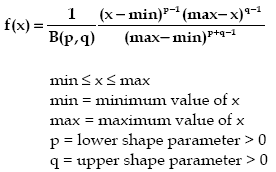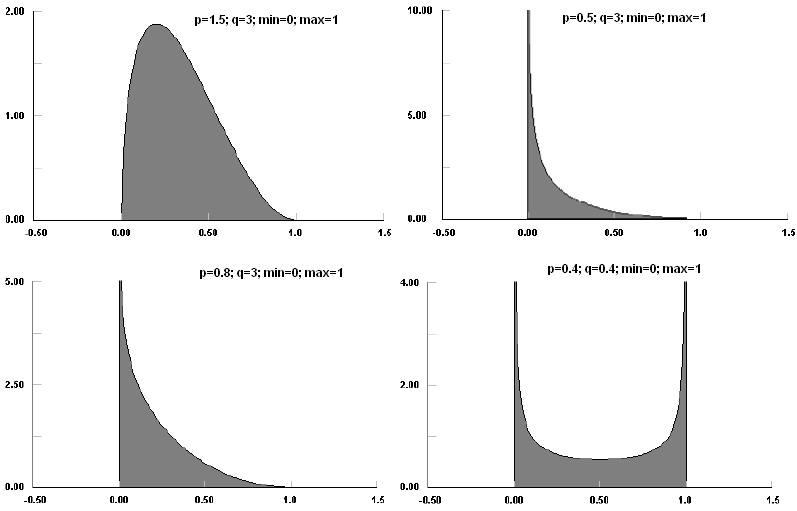AnyLogic

# betaThe Beta distribution is a continuous distribution that has both upper and lower finite bounds. Because many real situations can be bounded in this way, the Beta distribution can be used empirically to estimate the actual distribution before much data is available. Even when data is available, the Beta distribution should fit most data in a reasonable fashion, although it may not be the best fit. The Uniform distribution is a special case of the Beta distribution with p, q = 1.

The Beta distribution can approach zero or infinity at either of its bounds, with p controlling the lower bound and q controlling the upper bound. Values of p, q < 1 cause the Beta distribution to approach infinity at that bound. Values of p, q > 1 cause the Beta distribution to be finite at that bound.

Beta distributions have many, many uses. As summarized in Johnson et al., beta distributions have been used to model distributions of hydrological variables, logarithm of aerosol sizes, activity time in PERT analysis, isolation data in photo-voltaic system analysis, porosity or void ratio of soil, phase derivatives in communication theory, size of progeny in Escherchia Coli, dissipation rate in breakage models, proportions in gas mixtures, steady-state reflectivity, clutter, and power of radar signals, construction duration, particle size, tool wear, and others. Many of these uses occur because of the doubly bounded nature of the Beta distribution.### beta(double p, double q, double min, double max)

Description
Generates a sample of the Beta distribution.
Parameters
Name Type of value Description
p double The lower shape parameter > 0.
q double The upper shape parameter > 0.
min double The minimum x value.
max double The maximum x value.
Result
Type Description
double The generated sample.

### beta(double p, double q)

Description
Generates a sample of the Beta distribution with min set to 0 and max set to 1. Is equivalent to beta(p, q, 0, 1).
Parameters
Name Type of value Description
p double The lower shape parameter > 0.
q double The upper shape parameter > 0.
Result
Type Description
double The generated sample.

### beta(double p, double q, double min, double max, java.util.Random r)

Description
Generates a sample of the Beta distribution using the specified random number generator.
Parameters
Name Type of value Description
p double The lower shape parameter > 0.
q double The shape factor (positive integer).
min double The minimum x value.
max double The maximum x value.
r java.util.Random The random number generator.
Result
Type Description
double the generated sample

This document includes content from the “Stat::Fit User’s Manual”. Copyright 2016 Geer Mountain Software Corp.